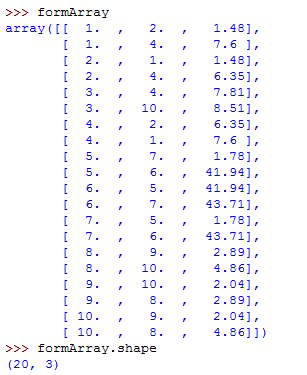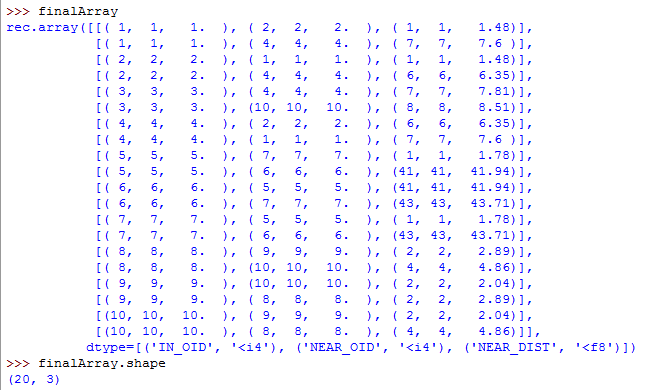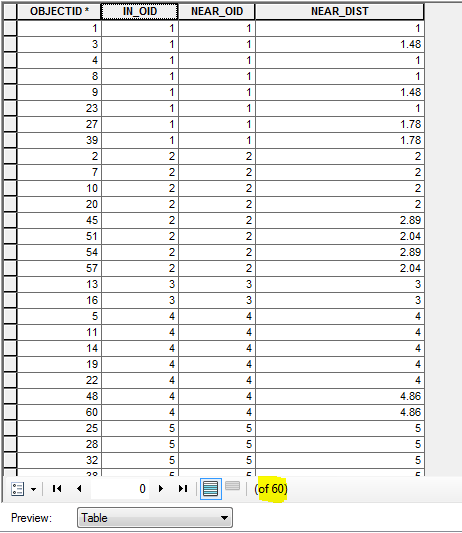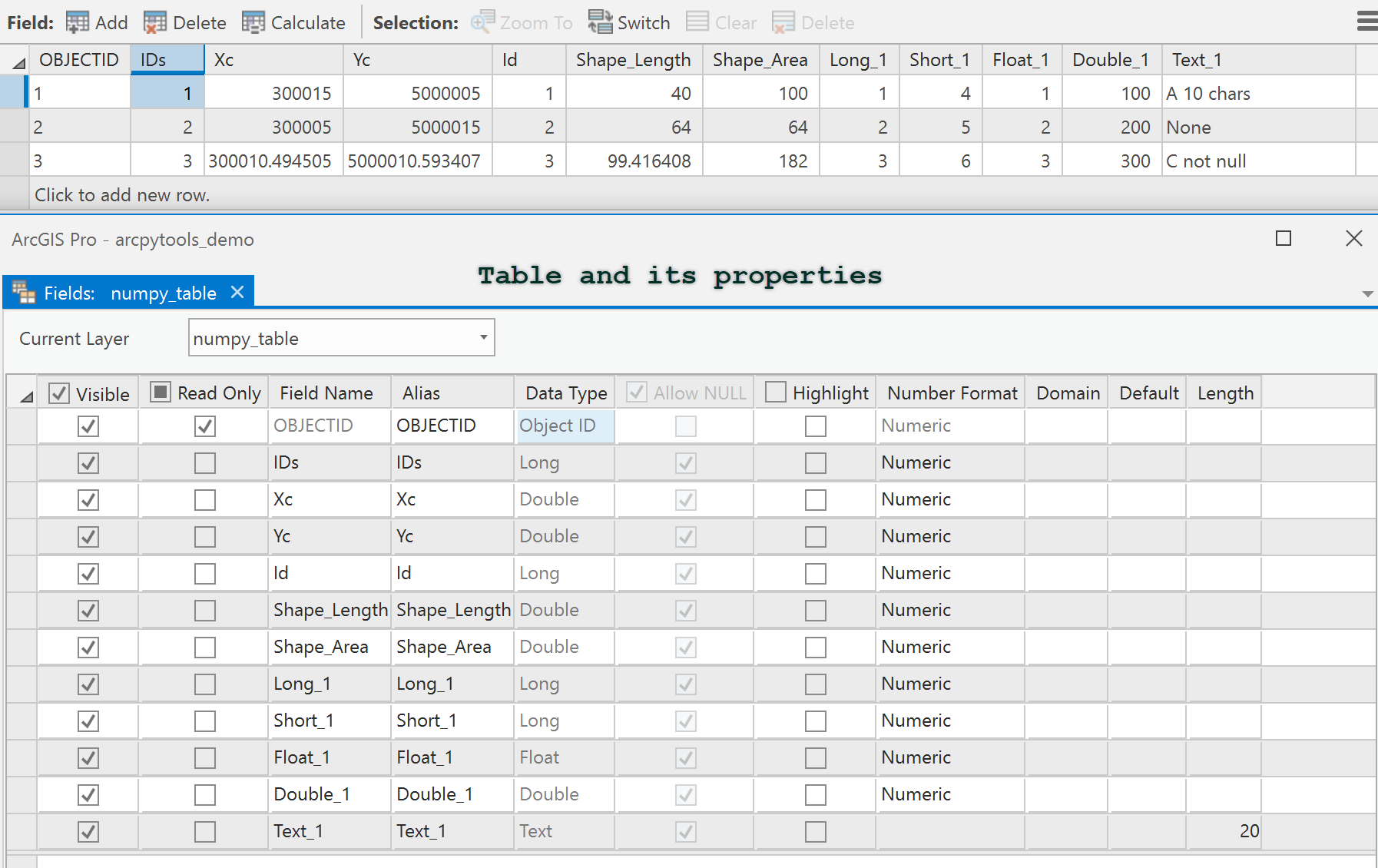# Numpy Array Gets Reshaped when Assigning Dtype

486
3
10-12-2017 01:45 PMbyMVP Regular Contributor

Essentially, I need to get a Numpy array (rows = x, cols = 3) to a geodatabase table.  What is happening is when I specify the dtype of the array to get the field names / types for the table, the array gets reshaped.

Any ideas?

``````#format array to create table from
#array of nearest values... reshaped to: rows=(inXY * toReturn), columns=3
formArray = numpy.array(listArray).reshape(-1,3)

dts = [('IN_OID', '<i4'),('NEAR_OID', '<i4'),('NEAR_DIST', '<f8')]

finalArray = numpy.rec.fromrecords(formArray, dtype = dts)

#export to geodatabase table
arcpy.da.NumPyArrayToTable(finalArray, r'...\ArcGIS\Default.gdb\out_table')‍‍‍‍‍‍‍‍‍‍‍‍‍‍‍‍‍‍‍‍``````

How the array structure should look:                                                 How the array looks after specifying dtypeAnd this is what the output table looks like (notice it has 60 rows... it should only contain 20)Tags (1)
3 RepliesbyMVP Legendary Contributor

Don't respecify the dtype.  your structured array (and recarrays) will have a dtype with a shape of (N, ) where N is the number of records.  You don't reshape a structured array to make it 'look' pretty, because adding that extra dimension will foul up your conversion to a table.  My Blog has dozens of posts on the use of numpy including some of the basics etc.  So what you had was fine until you reshaped it and added the extra dimension turning each row into 3byMVP Regular Contributor

Thanks Dan!  I need to somehow convert the first two columns of the array into integer types before converting to a table.  If not, the output table has 'Double' type in the ID fields.  Which is not ideal.  So that's why I tried to specify a dtype.  I guess I need to head to your blog and study arrays more.  Thanks again!byMVP Legendary Contributor

Mitch....

Observe (table and properties in Pro)

``````b
array([(1, 300015.0, 5000005.0, 1, 40.0, 100.0, 1, 4, 1.0, 100.0, 'A 10 chars'),
(2, 300005.0, 5000015.0, 2, 64.0, 64.0, 2, 5, 2.0, 200.0, 'None'),
(3, 300010.4945054945, 5000010.593406593, 3, 99.41640786499875, 182.0, 3, 6, 3.0, 300.0, 'C not null')],
dtype=[('IDs', '<i4'), ('Xc', '<f8'), ('Yc', '<f8'), ('Id', '<i4'),
('Shape_Length', '<f8'), ('Shape_Area', '<f8'), ('Long_1', '<i4'),
('Short_1', '<i4'), ('Float_1', '<f4'), ('Double_1', '<f8'),
('Text_1', '<U10')])

arcpy.da.NumPyArrayToTable(b, r"C:\Git_Dan\a_Data\testdata.gdb\numpy_table")‍‍‍‍‍‍‍‍‍‍``````

and in ProYou were working too hard, conversions and dtypes are converted to esri equivalents from numpy